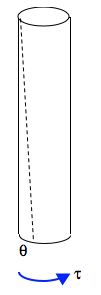$$\require{cancel}$$

22.4: Deducing Relationships

•• Contributed by Jeremy Tatum
• Emeritus Professor (Physics & Astronomy) at University of Victoria

i. We may suppose that the period $$P$$ of a simple pendulum depends upon its mass $$m$$, its length $$l$$, and the gravitational acceleration $$g$$. In particular we suppose that the period is proportional to some power $$\alpha$$ of the mass, some power $$\beta$$ of the length, and some power $$\gamma$$ of the gravitational acceleration. That is

$$p \propto m^\alpha l ^ \beta g ^ \gamma$$.

Both sides must have the same dimension - namely T.

That is

$$[ m^\alpha l ^ \beta g ^ \gamma ] = T$$

That is

$$\text{M}^\alpha \text{L}^\beta (\text{LT}^{-2}) ^\gamma = T$$

We equate powers of M, L and T to get three equations in $$\alpha, \beta, \gamma$$:

$$\alpha = 0, \beta + \gamma = 0, -2 \gamma = 1,$$

with solutions $$\alpha = 0, \beta = \frac{1}{2} , \gamma = - \frac{1}{2}$$ , which shows that

$$P \propto m^0l^{\frac{1}{2}} g^{-\frac{1}{2}}$$ , or $$P \propto \sqrt{\frac{l}{g}}$$

ii. Here’s another: The torque $$\tau$$ required to twist a solid metallic cylinder through an angle $$\theta$$ is proportional to $$\theta$$ : $$\tau = c \theta$$.$$c$$ is the torsion constant. How does $$c$$ depend upon the length $$l$$ and radius $$a$$ of the cylinder, its density $$\rho$$ and its shear modulus $$\eta$$ ? There is an immediate difficulty, in that we have four quantities to consider − $$l, a, \rho$$ and $$\eta$$, yet we have only three dimensions L, M , T to deal with. Hence we shall have three equations in four unknowns. Further, two of the quantities, $$l$$ and $$a$$ have similar dimensions, which adds to the difficulties.

In cases like this we may have to make a sensible assumption about one of the quantities. We may, for example, find it easy to accept that, the longer the cylinder, the easier it is to twist, and we may make the assumption that the torsion constant is inversely proportional to the first power of its length. Then we can suppose that

$$cl \propto a^\alpha \rho^\beta \eta^\gamma$$

in which case

$$[cl] \quad h \quad [ a^\alpha \rho^\beta \eta^\gamma]$$

That is

$$\text{ML}^2\text{T}^{-2}L \quad h \quad \text{L}^\alpha ( \text{ML}^{-3}) ^\beta (\text{ML}^{-1} \text {T}^ {-2}) ^\gamma$$

Equate the powers of M, L and T:

$$1 = \beta + \gamma; \qquad 3 = \alpha - 3 \beta - \gamma; \qquad -2 = -2\gamma$$

This gives $$\alpha = 4, \beta = 0, \gamma = 1,$$ and hence $$c \propto \frac{\eta a ^4}{l}$$.

iii. How does the orbital period $$P$$ of a planet depend on the radius of its orbit, the mass $$M$$ of the Sun, and the gravitational constant $$G$$?

Assume

$$P \propto G^\alpha M^\beta a^\gamma$$

It is left to the reader to show that $$P \propto \sqrt{\frac{a^3}{GM}}$$.

iv. A sphere of radius a moves slowly at a speed v through a fluid of density $$\rho$$ and dynamic viscosity $$\eta$$. How does the viscous drag $$F$$ depend upon these four variables?

Four variables, but only three dimensions, and hence three equations! What to do? If you have better insight than I have, or if you already know the answer, you can assume that it doesn’t depend upon the density. I haven’t got such clear insight, but I’d be willing to suppose that the viscous drag is proportional to the first power of the dynamic viscosity. In which case I’d be happy to assume that

$$\frac{F}{\eta} \propto a^\alpha \rho^\beta v^\gamma$$

Then

$$\frac{\text{MLT}^{-2} }{\text{ML}^{-1} \text{T}^{-1}} = \text{L}^\alpha (\text{ML}^{-3})^\beta ( \text{LT}^{-1} ) ^ \gamma$$

Equate the powers of M, L and T:

$$0 = \beta; \qquad 2 = \alpha - 3 \beta + \gamma' \qquad -1 = -\gamma$$

This gives $$\alpha = 1, \beta = 0, \gamma = 1$$ , and hence $$F \propto \eta a v$$.